# Dickson's conjecture

## Statement

Suppose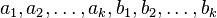are integers with all the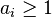. Then, consider the polynomials: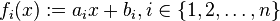Then, one of the following is true:

• There is a prime numbersuch that the product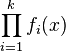istimes an integer-valued polynomial. In other words, one of the polynomials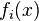is always congruent to 1 modulo.
• There exist infinitely many [[natural number]sfor which all the values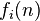are simultaneously prime.

## Related facts and conjectures

### Stronger facts and conjectures

• Schinzel's hypothesis H generalizes from linear polynomials to polynomial of arbitrary degree.
• Bateman-Horn conjecture further generalies Schinzel's hypothesis H by providing an asymptotic quantitative estimate of the frequency of occurrence of primes.